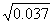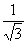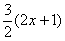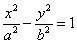Wednesday 21st October 2020CBSE Guess > Papers > Important Questions > Class XII > 2009 > Maths > Mathematics By Anil Kumar Tondak

CBSE CLASS XII

Application of Derivatives

Q. 1. The volume of a cube is increasing at a constant rate. Prove that the increase in surface area varies inversely as the length of the edge of the cube.

Q. 2.  Use differentials to find the approximate value ofQ. 3.  It is given that for the function f(x) = x03 – 6x2 + ax + b on [1, 3], Rolle’s theorem holds with
c = 2+. Find the values of a and b if f(1)= f(3) = 0

Q. 4. Find a point on the curve y = (x – 3)2, where the tangent is parallel to the line joining (4, 1)
and (3, 0).

Q. 5. Find the intervals in which the function f(x) = x4 – 8x3 + 22x2 – 24x + 21 is decreasing or increasing.

Q. 6. Find the local maximum or local minimum of the function.

f(x) = sin4x + cos4x,  0<x<p/2.

Q .7. Find the point on the curve y2 = 4x which is nearest to the point (2, 1).

Q. 8. A figure consists of a semi-circle with a rectangle on its diameter. Given the perimeter of the figure, find its dimensions in order that the area may be maximum.
Q. 9. A balloon which always remain spherical has a variable diameter. Find the rate of change of its volume with respect to x.

Q. 10. Find the intervals in which f(x) = (x+1)3 (x – 3)3 is strictly increasing or decreasing.

Q. 11. Prove that the curves x = y2  and xy = k cut at right angles if 8k2 = 1

Q. 12.  Using differentials, find the approximate value of (26.57)1/3

Q. 13. Show that of all the rectangles inscribed in a given fixed circle, the square has the maximum area.

Q. 14. Find the equation of the tangent and normal to the hyperbolaat the point (x0,y0)

Q. 15. Find the intervals of the function f(x) = sinx + cosx, 0£x£2p is strictly increasing or strictly decreasing.

Q. 16. An open topped box is to be constructed by removing equal squares from each corner of a 3 metre by 8 metre rectangular sheet of aluminium and folding up the sides. Find the volume of the largest such box.

Prepared By Anil Kumar Tondak
M.Sc.(Maths), M.Ed., M.A.(Eco),M.B.A.(Mkt)
Mob No. 9811363962

 Maths